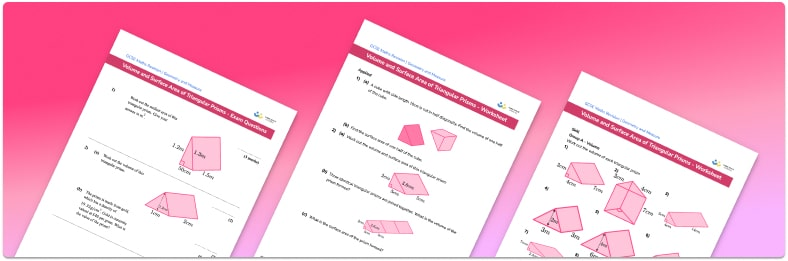# Volume And Surface Area Of A Triangular Prism WorksheetHelp your students prepare for their Maths GCSE with this free volume and surface area of triangular prisms worksheet of 44 questions and answers

• Section 1 of the volume and surface area of triangular prisms worksheet contains 36 skills-based volume and surface area of triangular prisms questions, in 3 groups to support differentiation
• Section 2 contains 4 applied volume and surface area of triangular prisms questions with a mix of worded problems and deeper problem solving questions
• Section 3 contains 4 foundation and higher level GCSE exam style volume and surface area of triangular prisms questions
• Answers and a mark scheme for all volume and surface area of triangular prisms questions are provided
• Questions follow variation theory with plenty of opportunities for students to work independently at their own level
• All questions created by fully qualified expert secondary maths teachers
• Suitable for GCSE maths revision for AQA, OCR and Edexcel exam boards

• This field is for validation purposes and should be left unchanged.

You can unsubscribe at any time (each email we send will contain an easy way to unsubscribe). To find out more about how we use your data, see our privacy policy.

### Volume and surface area of triangular prisms at a glance

Triangular prisms are 3d shapes consisting of two identical triangular faces at either end of the prism, connected by three rectangular faces.

These should not be confused with triangular pyramids as they do not have a constant cross-sectional area.

To find the volume of a triangular prism, we use the formula: Volume = area of cross-section ✖ length. As the cross-section of a triangular prism is a triangle, the height of the prism is identical to the height of the triangle. The volume formula works for any prism, including triangular prisms, rectangular prisms, L-shaped prisms, and trapezoidal prisms to name a few.

The volume of any 3d shape is measured in cubic units such as cubic centimetres (cm3), cubic metres (m3), or cubic millimetres (mm3).

The cross-section of a triangular prism is a triangle so to find its area we use the area formula for a triangle: Area equals a half, multiplied by the base, multiplied by the height. Area is measured in square units such as square centimetres (cm2), square metres (m2) or square millimetres (mm2).

To find the surface area of a triangular prism we calculate the area of each face. We then add these together to find the total surface area. Surface area (also called lateral surface area) is measured in square units. If a prism is standing upright, the cross sectional area we need to find is the area of the base of the prism. For example, a triangular prism standing on the cross sectional area would have a triangular base.

For example, take a right-angled triangular prism with the following side lengths: a base of b cm, a height of h cm, a slope length l cm, and a depth of d cm. The surface area of the triangular prism would be the area of the two right triangle faces, bh, added to the area of the other faces, d✖(b+h+l).

Looking forward, students can then progress to additional 3D shapes worksheets and more geometry worksheets for example an angles in polygons worksheet or volume and surface area of spheres worksheet.For more teaching and learning support on Geometry our GCSE maths lessons provide step by step support for all GCSE maths concepts.

## Do you have KS4 students who need more focused attention to succeed at GCSE?There will be students in your class who require individual attention to help them succeed in their maths GCSEs. In a class of 30, it’s not always easy to provide.

Help your students feel confident with exam-style questions and the strategies they’ll need to answer them correctly with our dedicated GCSE maths revision programme.

Lessons are selected to provide support where each student needs it most, and specially-trained GCSE maths tutors adapt the pitch and pace of each lesson. This ensures a personalised revision programme that raises grades and boosts confidence.

Find out more